There are all sorts of resonances around us, in the world, in our culture, and in our technology. A tidal resonance causes the 55 foot tides in the Bay of Fundy. Mechanical and acoustical resonances and their control are at the center of practically every musical instrument that ever existed. Even our voices and speech are based on controlling the resonances in our throat and mouth. Technology is also a heavy user of resonance. All clocks, radios, televisions, and gps navigating systems use electronic resonators at their very core. Doctors use magnetic resonance imaging or MRI to sense the resonances in atomic nuclei to map the insides of their patients. In spite of the great diversity of resonators, they all share many common properties. In this blog, we will delve into their various aspects. It is hoped that this will serve both the students and professionals who would like to understand more about resonators. I hope all will enjoy the animations.

Origins of Newton's laws of motion

History of mechanical clocks with animations
Understanding a mechanical clock with animations
includes pendulum, balance wheel, and quartz clocks

Saturday, June 23, 2018

Wave EquationsWave Equations

- what is required for an equation to be a wave equation? -

Mathematically we can define waves in several ways:

1. As a shifting sinusoid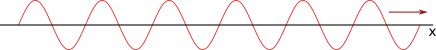mathematically expressed in real form as:    where A and B are real constants,     i.e. amplitudes,(1) or in complex form as:     where A is a complex amplitude,     i.e. an amplitude and phase shift.(2) In both the above forms κ is the wavenumber in units of radians per meter and ω is the angular frequency in units of radians per second. The independent variables x and t are position and time. In the complex form only the real part of the complex expression is considered to describe actual physical attributes of a wave. Because of this we are free to put a minus sign in front of the i in (2) without changing the physical attributes of the wave represented as long as we stay consistent in our use of a minus sign or not throughout the calculation at hand. Using a negative κ in either of the above equations represents waves propagating in the negative x direction.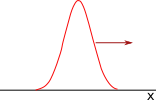2. A wave can also be an arbitrary function that keeps its shape and moves along as time progresses. You might notice that the sinusoids in (1) and (2) are functions of κxωt. Alternatively they could be written as functions of xct where c=ω/κ is the wave velocity. Note that κxωt can be factored as κxωt = κ×(xωt/κ) = κ×(xct) where κ is the wavenumber for the sinusoids of (1) and (2).

This method of using a function of xct can work for any arbitrary function. As an example at the right we see a Gaussian bell shaped function converted into a function that moves with velocity c by replacing the x with an x−ct.

 A stationary Gaussian as a function of x is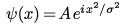.   (3) The moving Gaussian is(4) where σ is a measure of the width of the bell shaped curve. Note that we could have started with any function of x and made a moving version of that function by replacing all occurrences of x in that function with x−ct.

3. A different way to mathematically define a wave is with a differential equation which has as its solution functions such as those above. The most cited example of such a defining differential equation is "the wave equation":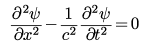.    (5)

The advantage of differential equations is that they allow the calculation of waves in different coordinate systems and varied boundary conditions such as for the waves inside a spherical resonator. Differential equations can also be modified to accommodate changing properties of the wave media such as varying wave velocities as a function of position.

Differential equations other than (5) may also serve as wave equations, even though (5) has become known as the official "wave equation".

In this posting we shall explore various differential equations and take a stab at what is required to be a general differential wave equation.

The wave equation - non-dispersive waves

Equation (5) has solutions which are non-dispersive, that is, waves whose velocity of propagation does not depend on the frequency of the wave. Examples of such waves are a acoustical waves of small amplitude and waves on a string, also of small amplitude. Another wave type which is non-dispersive is electromagnetic waves such as light, radio waves and x-rays. Such electromagnetic waves have a wave variable ( the ψ in (5) ) that is a vector in the general case; however the wave variable can be a scaler as in (5) in certain cases, the cases that are often treated in electromagnetic textbooks.

One property of a medium governed by a non-dispersive wave equation is that waves of arbitrary shape keep their shape as they propagate. One way to understand this is that any arbitrary function of x−ct is a solution to the wave equation (5). That is to say direct substitution of f(x−ct) into (5) maintains the equality shown in (5). This type of solution of "the wave equation" was first proposed by Jean le Rond d'Alembert in 1746.

To understand this we use the chain rule of differentiation:so that substituting ψ(x−ct) = ψ(u) into (5) yields:

where u=x−ct.

Another way to understand that waves of arbitrary shape propagate and maintain their shape is to understand that any function can be broken down into a sum of sinusoids of varying frequencies. Because these frequency components all travel at the same velocity, the shape will maintain itself.

In contrast in a medium with a dispersive wave equation, the various frequency components of an arbitrary shape will travel at different speeds and so add up differently as time progresses. This results in a wave that changes its shape as time progresses.

Another interesting exercise is to factor (5) which yields:

One solution of this equation occurs when the right most factor operating on ψ is zero:

Putting ψ = x−ct into the left side of (8) yields zero and thus satisfies (8), (7) and (5). Inverting the order of the two factors in (7) results in an equation like (8) but with a minus sign. That equation is satisfied by ψ = x+ct which is a sinusoidal wave traveling in the negative x direction.

We see that (5) is a second order differential wave equation with two solution: a positive going and a negative going wave. Equation (8) is a first order differential wave equation with only one solution, a positive going wave.

Water waves - dispersiveA common type of waves are those we see on the surface of water, i.e. water waves. These are dispersive. To better understand their dispersive nature we look at one of the defining differential equations, Equation (8) of this link.

We have replaced Δy of that link (the vertical displacement of the water's surface due to the wave) with δy. The quantity δx is the displacement of water in the x direction due to the passing wave.

Looking at Equations (3a) and (3b) of the the link we see that the ratio of δy/δx = −i tanh κy or solving for δy this is δy = −i δxtanh κy. Substituting this into (9) yields

where y is the depth of the water.

In (10) we have a wave equation expressing the horizontal displacement of the water parcels in response to a passing wave as a function of x and t.

To find out the constraint on these waves we substitute the complex traveling wave expression δx = A ei(κx−ωt) into (10) to get:  tanh κy = ω2 or:

We next solve for the phase velocity, i.e. the velocity of the wave crests. The standard equation for the phase velocity is vp = ω/κ which when used with (11) gives us:

Thus the phase velocity is not constant with frequency. Water waves are therefore dispersive, meaning that different frequencies travel at different velocities.

The group velocity, i.e. velocity of energy and pulses such as the Gaussian shown above, has the standard equation vg = /. Using (11) this yields:

Incidently, for waves which are without dispersion, i.e. all frequencies travel at the same speed as discussed in the previous section, the phase velocity equals the group velocity and both are a constant.

Waves in a waveguideMicrowaves (electromagnetic waves used for microwave ovens, cell phones and radar) are often channeled down metal pipes called waveguides. These have a rectangular cross section (width a and height b). While there are many modes of propagation, each with its own electric and magnetic field configuration, these modes can be lumped into two classes. We will focus on the TE modes which are the commercially most used. We will further restrict our study of TE modes with non-zero electric fields in the y direction, Ey.

The electromagnetic wave equation written for the Ey component of the electric field in free space is:

When confined to a metal waveguide the y electric field parallel to the walls at the walls must be zero. It is also true that Ey at the top and bottom of the waveguide must have ∂Ey/∂y = 0. Due to these two constraints the electric field must assume a solution in the form:

where the x and y wavenumbers are given by:

The modal indices m and n are positive integers. For the modes we are treating m must be greater than zero while n can be zero or greater. (The TE0,1 mode, i.e. m = 0, n = 1 mode, has all its electric field in the x direction and is zero in the y direction while the TE0,0 mode has all zero electromagnetic fields and is not a wave mode at all.)

Substituting (14) into (13a) yields:

The first two terms in (16) are set by the waveguide dimensions, i.e. by (15), and can be replaced by the cutoff frequence ωc given by:

Equation (17) calculates the lowest frequency that a particular mode will propagate in the z direction. We can use (17) with (16) to get:

Equation (18) is the defining differential wave equation for these fields propagating in the z direction. It is similar to (5) except that it has an additional term involving Ey times a constant (the first term in (18) ).

We next replace f(x,t) in (14) with the standard complex propagating sinusoid Aei(κzz−ωt) and then substitute the resulting Ey into (18). We finally solve for ω and get:

From this we can calculate the phase and group velocities:

Similar wave media: Electromagnetic waves in a plasma

A plasma consists of ionized gas molecules and free electrons and highly interacts with electromagnetic waves trying to propagate through it. There are a number of types of electromagnetic waves possible (with static magnetic fields or without and other criteria). See this link for details. One of the simpler cases is without a static magnetic field and is governed by this equation:

where ωp is the plasma frequency and is the lowest frequency at which this type of wave will propagate. It is related to the density of free electrons in the plasma. Alternate source: D.K.Cheng, 2nd Ed. Field and Wave Electromagnetics p.377 Eq.8-73. Equation (22) is basically the same as (19).

Similar wave media: the Klein Gordon Equation in quantum mechanics

The Klein Gordon equation governs the motion of spinless, chargeless particles which are moving at relativistic speeds (within an order of magnitude of the velocity of light):

where c is the velocity of light, m is the mass of the particles under study, and is a quantum mechanical constant.

With the standard complex propagating sinusoid Aei(κzz−ωt) substituted in for ψ this equation becomes:

Again we see the same dependence for the frequency ω on the wavenumber κ as we see in (19). All three waves have differential equations that have second order derivatives in space and time plus an extra term that is a constant times the wave parameter. All three display a minimum frequency of wave propagation, i.e. a cutoff frequency. All three approach dispersionless propagation at very high frequencies but show dispersion at frequencies just above the cutoff frequency.

Equation (24) is the relativistic equivalent of the Schrodinger equation which we will discuss next.

The Schrodinger equation

The Schrodinger equation governs the motion of spinless particles which are moving at non-relativistic speeds (much slower than the velocity of light):

where V is the potential the particle experiences and can vary with position.

With the standard complex propagating sinusoid Aei(κzz−ωt) substituted in for ψ this equation becomes:

In (26) we see a very different dispersion relation than we saw in the previous wave media. If potential V is zero, the frequency depends on the square of the wavenumber. If V is not zero but positive then there is an offset, and there will be a minimum propagating frequency; this occurs when a particle has sufficient energy (frequency and energy are proportional in quantum mechanics) similar to the cutoff frequencies in the previously discussed media.

We can calculate the phase and group velocitites: Equation (28) is consistent with the momentum p = mv = h/λ remembering that κ = 2π/λ and that ℏ = h/2π. The constant h is Planck's constant.

A comparison of the discussed wave types

Dispersion and wave velocitiesFig. 1. Comparison of the dispersion curves of the four wave types discussed above. We see that the "wave equation" or dispersionless curve is linear, that of the water wave shows its square root dependence, that of the wave guide approaches being linear at large wavenumbers, and that of the Schrodinger equation is parabolic. Fig. 2. Comparison of the phase velocities versus wavenumber of the four wave types discussed above. We see that the dispersionless curve is constant as expected, that of the water waves is largest at low wavenumbers (highest frequencies) and slowly decreases with increasing wavenumbers. The phase velocities of the wave guide waves and waves of the Schrodinger equation are infinite at low wavenumbers and become smaller at larger wavenumbers.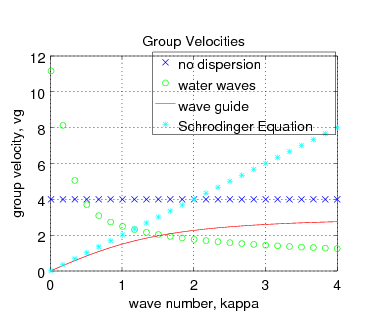Fig. 3. Group velocities for the four types of waves discussed above versus wavenumber. The "no dispersion" medium shows a group velocity equal to the phase velocity. The water waves have group velocities closely following the phase velocities. The wave guide shows group velocities related as the inverse of the phase velocities. The Schrodinger equation waves have group velocities linearly proportional to the wavenumber, that follows the quantum mechanics idea that momentum is proportional to the wavenumber.

Table 1. Differential wave equation types
No dispersion - the "wave equation"(30)
Water waves - waves on a liquid surface(31)

for large depth, i.e. κy >> 1 .

Waveguide(32)
The Schrodinger Equation(33)

The a's in the above equations are real constants. The differential equations (30) through (33) are taken from the preceding text with the constants replaced by a's.

Looking at the above differential wave equations in Table 1 we see that all the equations relate derivatives with respect to position x to derivatives with respect to time t. All the derivatives are operating on a wave parameter labelled ψ for the purposes of comparison.

What differs between the four equations are the derivative orders. That is, in (30) we see second derivatives with respect to both x and t whereas in (31) we see a first derivative with repect to x and second derivative with respect to t. Eqs. (32) and (33) have a third term which has no derivative.

Eqs. (31) and (33) have the complex constant i in them which signals that they are written for complex solutions. The i is also necessary so that the standard complex sinusoidal wave, i.e. Aei(κx−ωt), be a solution to the equation.

Requirements for a general differential wave equation

It would seem that we could write differential wave equations containing derivatives of any order, even fractional orders, as long as we included the required complex constant to insure that Aei(κx−ωt) be a solution.

Could we write a differential wave equation that did not have Aei(κx−ωt) as a solution but instead had some other solution that moved along in time? If that solution had a fixed shape as it moved along then this shape could be decomposed into sinusoidal Fourier components which would need to move along in unison so that the shape be preserved in time. This would indicate a non-dispersive differential equation of the type of (30) which of course has sinusoidal solutions.

So for a non-sinusoidal wave equation we are left with solutions which are non-sinusoidal and that do not maintain their shape as they moved along. Such "wave" solutions might be addressed by a differential equation which lacks the required complex constant to make Aei(κx−ωt) a solution. It is not clear that these would be classified as waves.

Example of creating a diferential wave equation

Suppose we want to create a differential wave equation for a media that supports sinusoidal waves and has a dispersion relation of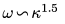. To avoid fractional order derivatives we square both sides to have integer powers of κ and ω:where a is the proportionality constant. We now write a differential equation that includes a second derivative of ψ with respect to time t and a third derivative with respect to position x:where b is a complex constant to be determined. We substitute our standard wave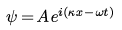into that and arrive at:. This meansor. Substituting b into our differential equation we have our final wave equation:.

Non-linear wave equation?

All the above work assumes that we are dealing with a linear wave medium. That is to say if ψ1 and ψ2 are both solutions of the wave equation for the medium in question then the sum of these, i.e. ψ3 = ψ1 + ψ2, will also be a solution. Note for example that our derived wave equation just above is first order in ψ even though it involves higher order derivatives of ψ.

In reality, many wave media are not completely linear, especially for large amplitude waves. We will not address such waves here, except to say they are more complicated to deal with.